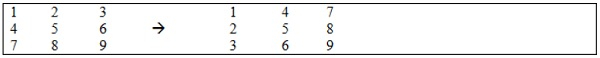# Swap Upper diagonal with Lower in C++

This tutorial is designed to swap the upper row of a three-diagonal array to its lower one using c++ code. Moreover, if a three-diagonal array is an input, the coveted results must be something like that as;For this, the course of action is briefed in the algorithm as follows;

## Algorithm

Step-1: Input a diagonal array
Step-2: Pass it to Swap() method
Step-3: Traverse the outer loop till 3
Step-4: increment j= i+ 1 in the inner loop till 3
Step-5: put the array value in a temp variable
Step-6: interchange the value arr[i][j]= arr[j][i]
Step-7: put the temp data to arr[j][i]
Step-8: Print using for loop

So, the c++ code is drafted as following in the consonance of the aforesaid algorithm;

## Example

Live Demo

#include <iostream>
#define n 3
using namespace std;
// Function to swap the diagonal
void swap(int arr[n][n]){
// Loop for swap the elements of matrix.
for (int i = 0; i < n; i++) {
for (int j = i + 1; j < n; j++) {
int temp = arr[i][j];
arr[i][j] = arr[j][i];
arr[j][i] = temp;
}
}
// Loop for print the matrix elements.
for (int i = 0; i < n; i++) {
for (int j = 0; j < n; j++)
cout << arr[i][j] << " ";
cout << endl;
}
}
// Driver function to run the program
int main(){
int arr[n][n] = {
{ 1, 2, 3},
{ 4, 5, 6},
{ 7, 8, 9},
};
cout<<"Input::"<<endl;
for (int i = 0; i < n; i++) {
for (int j = 0; j < n; j++)
cout << arr[i][j]<< " ";
cout << endl;
}
// Function call
cout<<"Output(Swaped)::"<<endl;
swap(arr);
return 0;
}

## Output

As seen below in the output, the swapped upper row with the lower segment of a 3D array as;

Input::
1 2 3
4 5 6
7 8 9
Output(Swaped)::
1 4 7
2 5 8
3 6 9